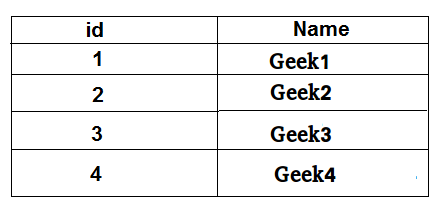Related Articles

# IFNULL in MySQL

• Last Updated : 03 Mar, 2018

Given a TABLE, in this TABLE, it prints entry of the table. If table is empty then it gives NULL.

Examples:

QUESTION : Given an employee table, print name from the given table which id equals to 2.Output : Geek2

QUESTION : Given same Employee table, print name from the given table which id equals to 5.
Output : NULL

Approach: In this case, we use here IFNULL. IFNULL print the null if the table is an empty or other condition.

Query:-

```SELECT
IFNULL(
(SELECT NAME
from employee
where id = 2),
'NULL') as NAME;
```
```Output:-
Geek2
```

Query:-

```SELECT
IFNULL(
(SELECT NAME
from employee
where id = 5),
'NULL') as NAME;
```
```Output:-
NULL
```
My Personal Notes arrow_drop_up